# 10-327/Classnotes for Thursday November 4

See some blackboard shots at BBS/10_327-101104-142342.jpg.

 Dror's notes above / Student's notes below
• Question: Regarding the proof of the Lebesque Number Lemma, let T(x) be the function we were working with in the proof. I am confused with how we reached the conclusion that if d(x,y)<E, then T(y)>= T(x) - E. I know that it was said that this is just an application of the triangle inequality, but I am having a bit of trouble seeing that. Hopefully someone can make this point a bit clearer for me. Thanks! Jason.
• If you could find a ball of radius 7 around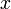$x$ which fits inside some set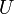$U$, and you move$x$ just a 1 unit away to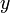$y$, then by the triangle inequality the ball of radius 6 around$y$ is entirely contained inside the ball of radius 7 around$x$ so it is entirely contained in$U$. Drorbn 18:37, 6 November 2010 (EDT)
• I have some doubts with Lebesgue number lemma too.. this delta(x) isn't a radius that we can fit a ball inside one of the U's. It is the supremum of all possible radius. Wouldn't that give us a problem? Don't we need to subtract some small positive value and then find a valid radius? I am not sure if there should be some technicality involved here.-Kai

And once we found this delta(x_0) we should divide by 2 so that delta(x)>delta(x_0) for all x in X? Because it is not necessarily true that we can find a ball of radius delta(x_0) around x_0 to fit into ball?-Kai

• I think you are correct, (if you follow the proof on the blackboard shots to the letter) as an example of what you are talking about take the open cover of [0,1] using sets of the form [0,x) for x<3/4 and (y,1] for y>1/4. Then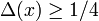$\Delta (x)\geq 1/4$ and at 1/2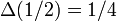$\Delta (1/2)=1/4$ but no ball of radius 1/4 around 1/2 fits inside any of the sets. So we will need to take a smaller value than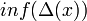$inf(\Delta (x))$ as our value of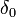$\delta_0$ for Lebesgue's Lemma. Dividing by two as you suggest should work fine to fix this problem... Maybe it's just me, but in proof's like this I always feel the urge to divide the final answer by 2, just in case I mixed up some some strict inequality, with a non-strict one somewhere - John
• Thanks John very nice example. Can you also help me with this question? "delta(x) isn't a radius that we can fit a ball inside one of the U's. It is the supremum of all possible radius. Wouldn't that give us a problem in showing delta(y)>=delta(x)-epsilon when d(x,y)<epsilon?"-Kai
• I don't think there is any problem with this step.

Without loss of generality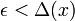$\epsilon < \Delta (x)$ otherwise the condition holds vacuously. Suppose we construct a ball of radius r such that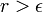$r > \epsilon$ around x so that it is a subset of some U in the cover, then we can construct a ball of radius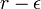$r - \epsilon$ around y such that this ball is also in U. So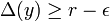$\Delta (y) \geq r-\epsilon$ for all r such that a ball of radius r exists around x as in the proof. Which implies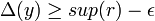$\Delta (y) \geq sup(r) - \epsilon$ implies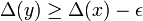$\Delta (y) \geq \Delta (x) - \epsilon$. Hopefully this makes sense and works - John -Yes. Makes sense. Nice and concise thanks! -Kai